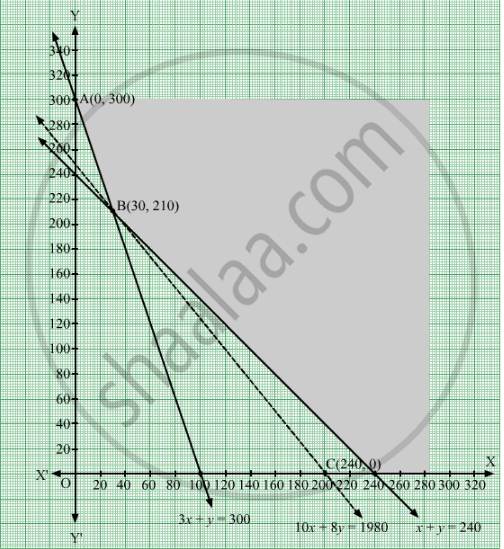# There Are Two Types of Fertilisers 'A' and 'B' . 'A' Consists of 12% Nitrogen and 5% Phosphoric Acid Whereas 'B' Consists of 4% Nitrogen and 5% Phosphoric Acid. - Mathematics

Sum

There are two types of fertilisers 'A' and 'B' . 'A' consists of 12% nitrogen and 5% phosphoric acid whereas 'B' consists of 4% nitrogen and 5% phosphoric acid. After testing the soil conditions, farmer finds that he needs at least 12 kg of nitrogen and 12 kg of phosphoric acid for his crops. If 'A' costs ₹10 per kg and 'B' cost ₹8 per kg, then graphically determine how much of each type of fertiliser should be used so that nutrient requiremnets are met at a minimum cost

#### Solution

The given information can tabulated as follows:

 Fertilizer Nitrogen Phosphoric Acid Cost/kg (in ₹) A 12% 5% 10 B 4% 5% 8

Let the requirement of fertilizer A by the farmer be x kg and that of B be y kg.
It is given that farmer requires atleast 12 kg of nitrogen and 12 kg of phosphoric acid for his crops.
The inequations thus formed based on the given information are as follows:

$\frac{12}{100}x + \frac{4}{100}y \geq 12$
$\Rightarrow 12x + 4y \geq 1200$
$\Rightarrow 3x + y \geq 300 . . . . . \left( 1 \right)$

Also,

$\frac{5x}{100} + \frac{5y}{100} \geq 12$
$\Rightarrow 5x + 5y \geq 1200$
$\Rightarrow x + y \geq 240 . . . . . \left( 2 \right)$

Total cost of the fertilizer Z = ₹ (10x + 8y)
Therefore, the mathematical formulation of the given linear programming problem can be stated as:
Minimize Z = 10x + 8
Subject to the constraints
3x + y ≥ 300         .....(1)
x + y  ≥ 240          .....(2)
x ≥ 0, y ≥ 0           .....(3)
The feasible region determined by constraints (1) to (3) is graphically represented as:Here, it is seen that the feasible region is unbounded. The values of Z at the corner points of the feasible region are represented in tabular form as:

 Corner Point Z = 10x + 8y A(0, 300) Z = 10 × 0 + 8 × 300 = 2400 B(30, 210) Z = 10 × 30 + 8 × 210 = 1980 C(240, 0) Z = 10 × 240 + 8 × 0 = 2400

The open half plane determined by 10x + 8y < 1980 has no point in common with the feasible region. So, the minimum value of Z is 1980.
The minimum value of Z is 1980, which is obtained at x = 30 and y = 210.
Thus, the minimum requirement of fertilizer of type A will be 30 kg and that of type B will be 210 kg.
Also, the total minimum cost of the fertilisers is ₹ 1980.

Concept: Graphical Method of Solving Linear Programming Problems
Is there an error in this question or solution?

#### APPEARS IN

RD Sharma Class 12 Maths
Chapter 30 Linear programming
Exercise 30.4 | Q 54 | Page 57